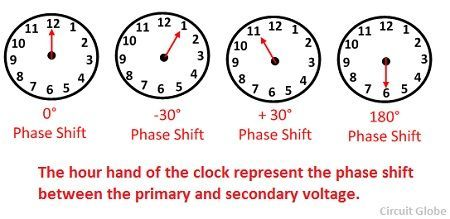# Transformer Vector Groups

Definition: The transformer vector group show the phase difference between the primary and secondary sides of the transformer. It also determines the high voltage and low voltage windings arrangement of three phase transformers.The three phase transformer is connected in various ways. On the basis of connection, the vector group of the transformer is determined.

Three phase transformer is divided into four main groups according to the phase difference between the corresponding line voltage on the high voltage side and the low voltage sides. The phase difference is the angle by which the low voltage line lags the high line voltage, and is measured in units of 30° in clockwise direction. These groups are

• Group number 1 – no phase displacement
• Group number 2 – 180° phase displacement.
• Group number 3 – (-30°) phase displacement.
• Group number 4 – (+ 30°) phase displacement.

The connection Y d 11 gives the following information – Y indicates that the high voltage is connected to star and d indicates the low voltage is connected in delta. The 11 indicates that the low line voltage lag, high line voltage by 11 Χ 30° = 330° measured from higher voltage phasor in a clockwise direction.

The phasor differences can also be measured by using the clock methods. Consider the minute hand of the clock shown the high voltage and the low voltage winding is represented by the hour hand. The angle of 30° is the angle between two adjacent figures on the clock dial and is taken as the unit of dial shift.When the hour hand of the clock is at 12, then the phase displacement is zero. When the hour hand is at 1 then the phase shift -30° degree. At 6 the phase shift is 6 Χ 30º = 180º. Similarly, when the hour hand is at 11 the phase shift is 11 Χ 30º  = 330º.

The number 0, 6, 1, and 11 in the group reference number indicates the primary to secondary phase shift regarding the hours of the clock. The connection designated by D y 11 is the delta-star transformer in which the low voltage line phasor is at 11 and is a phase advanced of +30° on the corresponding line voltage on the high voltage side.

Note: The only transformer in the same group may be connected in parallel. For example, a star-star, 3-phase transformers can be parallel with another three phase transformer whose windings are either connected in Y-Y or ∆-∆. The ∆-∆ transformer cannot be parallel with Y-∆ transformer.

### 2 thoughts on “Transformer Vector Groups”

1. Sabapathy Sivaraman

Very simply explained such that the mechanical engineer like me also could understand it.

Thanks a lot